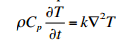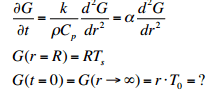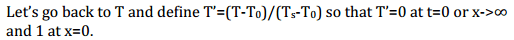# Normalizing boundary conditions

Hi All,

This is my first post on these forums. I am not looking for a solution to this problem but more interested in seeing if someone can point me to a resource that can explain the following. Thanks in advance for any help.

I'm trying to solve a pde which gives a temperature profile.We end up changing over to spherical coordinates where our boundary conditions are not completely clear although our initial condition is.The professor writes something like this. basically it normalizes the boundary conditions to (0,1). I can't wrap my head around this and googling for a while hasn't given me any results that clarify this.Thanks for the post! This is an automated courtesy bump. Sorry you aren't generating responses at the moment. Do you have any further information, come to any new conclusions or is it possible to reword the post?

pasmith
Homework Helper
The professor writes something like this. basically it normalizes the boundary conditions to (0,1). I can't wrap my head around this and googling for a while hasn't given me any results that clarify this.

View attachment 75728

This is a straightforward linear rescaling of the form $$f : [a,b] \to [0,1] : x \mapsto \frac{x - a}{b - a}.$$ Observe that $f$ is strictly increasing with $f(a) = 0$ and $f(b) = 1$.

If what you are struggling with is "why does he bother to do this?" then the concept you are looking for is nondimensionalization. Also it's easier to work with $G(t = 0) = G(r \to \infty) = 0$ rather than $G(t = 0) = rT_0$ and $G(r \to \infty) = \infty$.